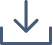### Gaussian Processes for Modelling the Navier Stokes Equations

The Navier Stokes equations are a system of DEs used to model fluid dynamics. In Western Australia, they are used to model the vibrations of underwater pipes. However, in this context, they do not have an analytical solution.

Numerical solutions can be found, however, this requires a supercomputer, and 6 weeks to evaluate 9 points. Therefore, given a limited number of points, we want to be able to interpelate between them. To do this, we will consider the Gaussian process.

For this research project, I will prove the convergence of the Gaussian process, and write python code to apply it to the points numerically calculated using a supercomputer. Furthermore, if time allows, I will study the inverse problem, where given “noisy” data, to solve for input values. Additionally, if time allows, I will study the experimental design problem – determining the optimal points to numerically solve for such that the solution can be best approximated.#### Elizabeth Mabbutt

University of Wollongong

Elizabeth (Liz) Mabbutt is a 2nd year Bachelor of Mathematics student at the University of Wollongong. She has been interested in statistics since taking an introduction to statistics course in first year, and has also developed an interest in pure mathematics and an enjoyment of writing proofs since taking a real analysis course in second year. She is looking towards starting honours in 2025 and a PhD afterwards.

Liz participated in a 2-week research scholarship in the winter of 2023 focusing on metric spaces and a proof of Hutchinson’s Theorem, where she enjoyed collaborating with other people and learning new concepts. She is looking forward to doing more research in other areas of mathematics she is interested in.